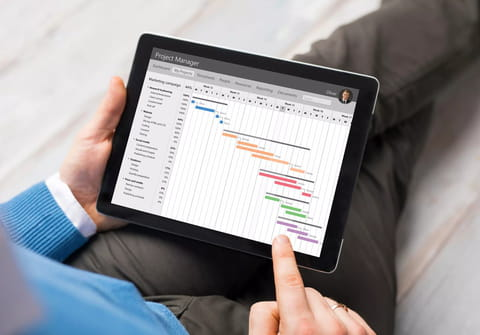# Transfer Excel data from one sheet to another: VBAIn this article, we will provide a VBA code to transfer data from one Excel sheet to multiple others while respecting specific conditions.

excel-sheet-to-another">To transfer data from one Excel sheet to another:

You can use following codes

```Sub SplitSheets()
Dim DataSht, wsCrit, SplitSht As Worksheet
Dim lrUnq, lrData, i As Long
Dim FtrVal As String
Application.ScreenUpdating = False
Set DataSht = Worksheets("sheet1") 'change it to the name of your raw data sheet
lrData = DataSht.Range("a" & Rows.Count).End(xlUp).Row
CopyToRange:=wsCrit.Range("A1"), Unique:=True
lrUnq = wsCrit.Range("a" & Rows.Count).End(xlUp).Row
For i = 2 To lrUnq
FtrVal = wsCrit.Range("A" & i).Value
DataSht.Select
'DataSht.ShowAllData
ActiveSheet.AutoFilterMode = False
ActiveSheet.Range("A1:Z" & lrData).AutoFilter Field:=2, Criteria1:=FtrVal
Range("a1").Select
Range(Selection, Selection.End(xlToRight)).Select
Range(Selection, Selection.End(xlDown)).Select
Selection.Copy
SplitSht.Select
Range("A1").Select
ActiveSheet.Paste
'Cells.Select
Cells.EntireColumn.AutoFit
SplitSht.Name = FtrVal
Application.CutCopyMode = False
Next i